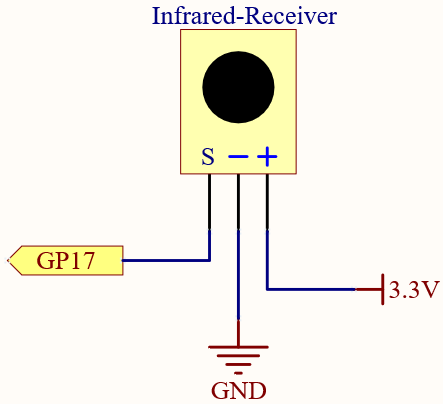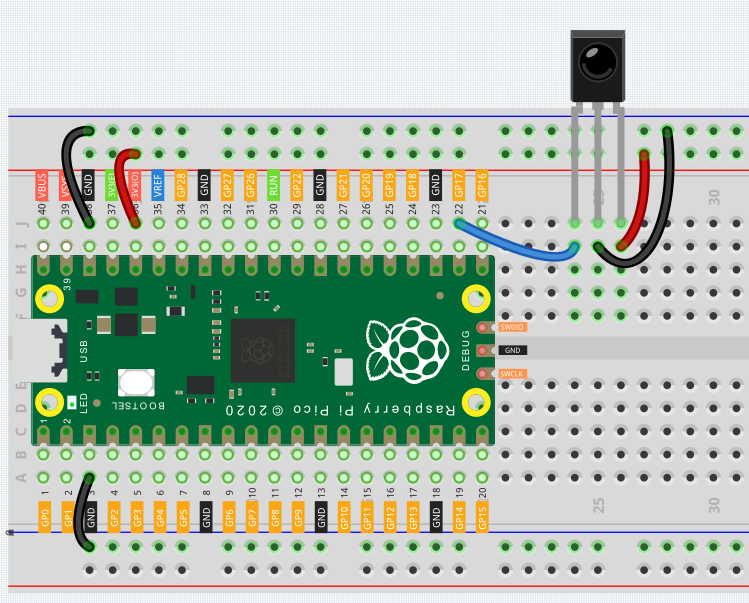# 6.4 IR Remote Control¶

In consumer electronics, remote controls are used to operate devices such as televisions and DVD players. In some cases, remote controls allow people to operate devices that are out of their reach, such as central air conditioners.

IR Receiver is a component with photocell that is tuned to receive to infrared light. It is almost always used for remote control detection - every TV and DVD player has one of these in the front to receive for the IR signal from the clicker. Inside the remote control is a matching IR LED, which emits IR pulses to tell the TV to turn on, off or change channels.

SchematicWiringCode

Note

• Open the `6.4_ir_remote_control.py` file under the path of `euler-kit/micropython` or copy this code into Thonny, then click “Run Current Script” or simply press F5 to run it.

• Don’t forget to click on the “MicroPython (Raspberry Pi Pico)” interpreter in the bottom right corner.

• For detailed tutorials, please refer to Open and Run Code Directly.

• Here you need to use the libraries in `ir_rx` folder, please check if it has been uploaded to Pico, for a detailed tutorial refer to Upload the Libraries to Pico.

```import time
from machine import Pin, freq
from ir_rx.print_error import print_error
from ir_rx.nec import NEC_8

pin_ir = Pin(17, Pin.IN)

def decodeKeyValue(data):
if data == 0x16:
return "0"
if data == 0x0C:
return "1"
if data == 0x18:
return "2"
if data == 0x5E:
return "3"
if data == 0x08:
return "4"
if data == 0x1C:
return "5"
if data == 0x5A:
return "6"
if data == 0x42:
return "7"
if data == 0x52:
return "8"
if data == 0x4A:
return "9"
if data == 0x09:
return "+"
if data == 0x15:
return "-"
if data == 0x7:
return "EQ"
if data == 0x0D:
return "U/SD"
if data == 0x19:
return "CYCLE"
if data == 0x44:
return "PLAY/PAUSE"
if data == 0x43:
return "FORWARD"
if data == 0x40:
return "BACKWARD"
if data == 0x45:
return "POWER"
if data == 0x47:
return "MUTE"
if data == 0x46:
return "MODE"
return "ERROR"

# User callback
if data < 0:  # NEC protocol sends repeat codes.
pass
else:
print(decodeKeyValue(data))

ir = NEC_8(pin_ir, callback)  # Instantiate receiver
ir.error_function(print_error)  # Show debug information

try:
while True:
pass
except KeyboardInterrupt:
ir.close()
```

The new remote control has a plastic piece at the end to isolate the battery inside. You need to pull out this plastic piece to power up the remote when you are using it. Once the program is running, when you press the remote control, the Shell will print out the key you pressed.

How it works?

This program looks slightly complicated, but it actually does the basic functions of the IR receiver with just a few lines.

```import time
from machine import Pin, freq
from ir_rx.nec import NEC_8

pin_ir = Pin(17, Pin.IN)

# User callback
if data < 0:  # NEC protocol sends repeat codes.
pass
else:
print(decodeKeyValue(data))

ir = NEC_8(pin_ir, callback)  # Instantiate receiver
```

Here an `ir` object is instantiated, which reads the signals acquired by the IR receiver at any time.

The result will be recorded in `data` of the callback function.

If the IR receiver receives duplicate values (e.g. pressing a key and not releasing it), then data < 0 and this data needs to be filtered.

Otherwise data would be a usable value, but some unspeakable code, and the `decodeKeyValue(data)` function is used to decode it.

```def decodeKeyValue(data):
if data == 0x16:
return "0"
if data == 0x0C:
return "1"
if data == 0x18:
return "2"
if data == 0x5E:
return "3"
if data == 0x08:
return "4"
if data == 0x1C:
return "5"
if data == 0x5A:
return "6"
if data == 0x42:
return "7"
if data == 0x52:
return "8"
if data == 0x4A:
return "9"
if data == 0x09:
return "+"
if data == 0x15:
return "-"
if data == 0x7:
return "EQ"
if data == 0x0D:
return "U/SD"
if data == 0x19:
return "CYCLE"
if data == 0x44:
return "PLAY/PAUSE"
if data == 0x43:
return "FORWARD"
if data == 0x40:
return "BACKWARD"
if data == 0x45:
return "POWER"
if data == 0x47:
return "MUTE"
if data == 0x46:
return "MODE"
return "ERROR"
```

If we press key 1, the IR receiver outputs a value like `0x0C`, which needs to be decoded to correspond to the specific key.

Next are some debug functions. They are important, but not related to the effect we need to achieve, so we just put them in the program.

```from ir_rx.print_error import print_error

ir.error_function(print_error) # Show debug information
```

Finally, we use an empty loop as the main program. And use try-except to make the program exit with the `ir` object terminated.

```try:
while True:
pass
except KeyboardInterrupt:
ir.close()
```